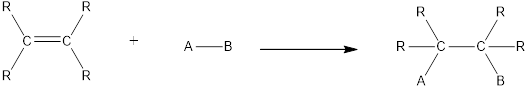Chapter 2, Problem 2.76EP### Organic And Biological Chemistry

7th Edition
STOKER + 1 other
ISBN: 9781305081079

#### Solutions

Chapter
Section### Organic And Biological Chemistry

7th Edition
STOKER + 1 other
ISBN: 9781305081079
Textbook Problem

# Write a chemical equation showing reactants, products, and catalysts needed (if any) for the reaction of propene with each of the reactants in Problem 13-72. Use Markovnikov’s rule as needed.

(a)

Interpretation Introduction

Interpretation:

Chemical equation that shows reactants, product and catalyst needed for the reaction of propene with water based on Markovnikov’s rule has to be written.

Concept Introduction:

Chemical reaction in which an atom or a group of atoms are added to each carbon atom of a carbon‑carbon multiple bond in a hydrocarbon or hydrocarbon derivative is known as addition reaction.

In this reaction no atoms or group of atoms are removed. Instead the unsaturated bond is reduced to saturated bond. A general scheme for addition reaction of alkene can be given as shown below,Asymmetrical addition reaction is the one in which two different atoms or group of atoms are substituted across the multiple bond resulting in the formation of product. No catalyst is required for this reaction.

Explanation

Propene reacts with water to give alcohol as product. This is an example of asymmetrical addition reaction. Hydration of propene using water results in the formation of 2-2-propanol as product. For this reaction no catalyst is required...

(b)

Interpretation Introduction

Interpretation:

Chemical equation that shows reactants, product and catalyst needed for the reaction of propene with bromine based on Markovnikov’s rule has to be written.

Concept Introduction:

Chemical reaction in which an atom or a group of atoms are added to each carbon atom of a carbon‑carbon multiple bond in a hydrocarbon or hydrocarbon derivative is known as addition reaction.

In this reaction no atoms or group of atoms are removed. Instead the unsaturated bond is reduced to saturated bond. A general scheme for addition reaction of alkene can be given as shown below,Halogenation reaction is an example of addition reaction. In this reaction, the halogen atoms are added across the double bonds. Chlorination and bromination are the most commonly used halogenation reaction. For halogenation reaction, no catalyst is required.

Markovnikov’s rule:

When an unsymmetrical molecule of formula HQ to an unsymmeterical alkene, the hydrogen atom from HQ gets attached to the unsaturated carbon atom which has the most hydrogen atoms. In other words, it can be said that the hydrogen atom gets attached to the unsaturated carbon atom that is least substituted.

(c)

Interpretation Introduction

Interpretation:

Chemical equation that shows reactants, product and catalyst needed for the reaction of propene with hydrogen iodide based on Markovnikov’s rule has to be written.

Concept Introduction:

Chemical reaction in which an atom or a group of atoms are added to each carbon atom of a carbon‑carbon multiple bond in a hydrocarbon or hydrocarbon derivative is known as addition reaction.

In this reaction no atoms or group of atoms are removed. Instead the unsaturated bond is reduced to saturated bond. A general scheme for addition reaction of alkene can be given as shown below,Asymmetrical addition reaction is the one in which two different atoms or group of atoms are substituted across the multiple bond resulting in the formation of product. No catalyst is required for this reaction.

(d)

Interpretation Introduction

Interpretation:

Chemical equation that shows reactants, product and catalyst needed for the reaction of propene with iodine based on Markovnikov’s rule has to be written.

Concept Introduction:

Chemical reaction in which an atom or a group of atoms are added to each carbon atom of a carbon‑carbon multiple bond in a hydrocarbon or hydrocarbon derivative is known as addition reaction.

In this reaction no atoms or group of atoms are removed. Instead the unsaturated bond is reduced to saturated bond. A general scheme for addition reaction of alkene can be given as shown below,Halogenation reaction is an example of addition reaction. In this reaction, the halogen atoms are added across the double bonds. Chlorination and bromination are the most commonly used halogenation reaction. For halogenation reaction, no catalyst is required.

Markovnikov’s rule:

When an unsymmetrical molecule of formula HQ to an unsymmeterical alkene, the hydrogen atom from HQ gets attached to the unsaturated carbon atom which has the most hydrogen atoms. In other words, it can be said that the hydrogen atom gets attached to the unsaturated carbon atom that is least substituted.

### Still sussing out bartleby?

Check out a sample textbook solution.

See a sample solution

#### The Solution to Your Study Problems

Bartleby provides explanations to thousands of textbook problems written by our experts, many with advanced degrees!

Get Started